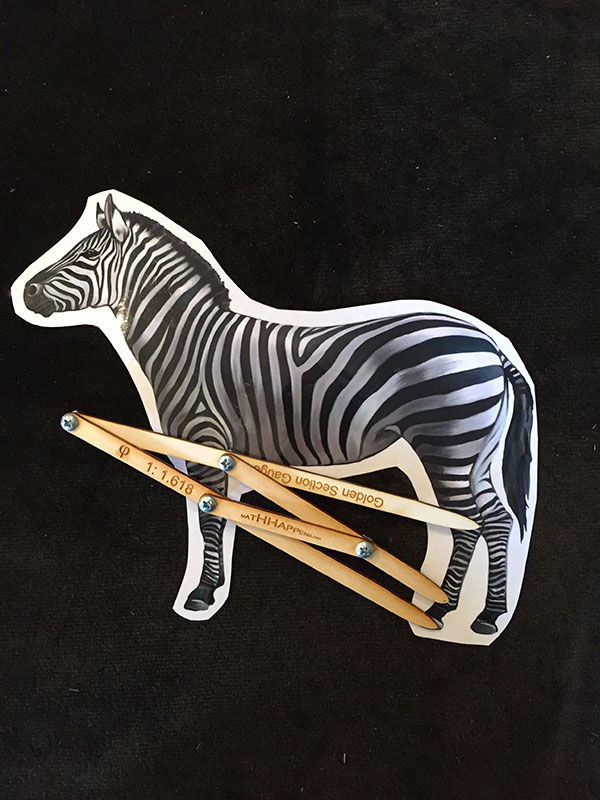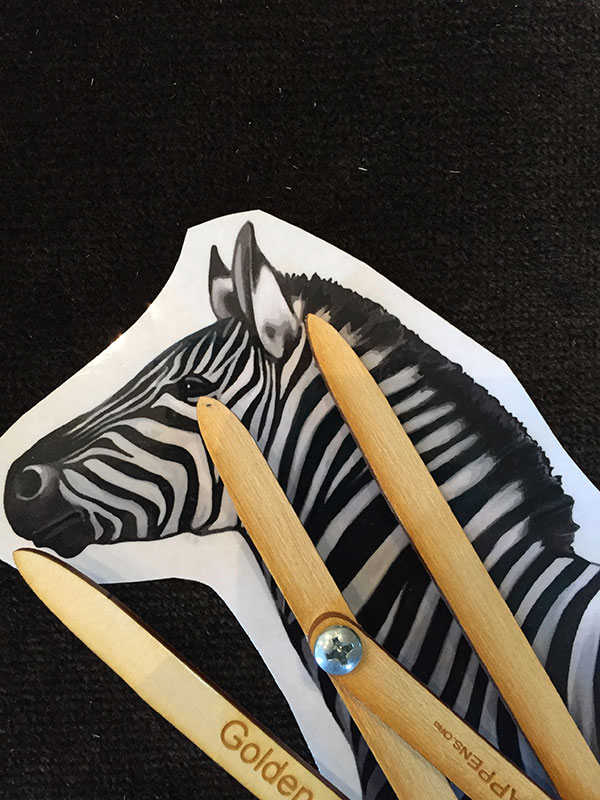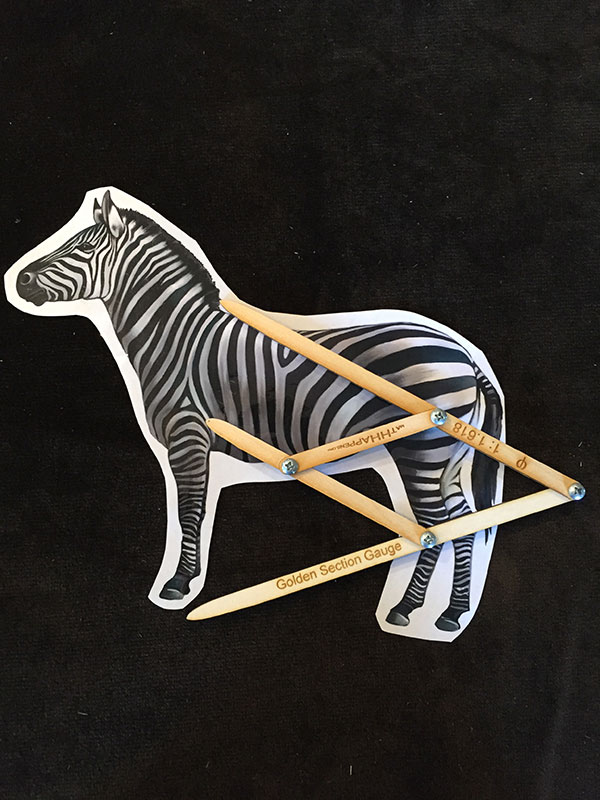# Golden Ratio

The Golden Ratio (Phi) is an irrational number. It has decimal digits that go on forever! Pi has this same characteristic. Pi can be found in the ratio of the circumference to the diameter of any perfect circle. The Golden Ratio or Phi can be found in the ratio of the arm of a five pointed star to the side of the pentagon at its center. Phi is a number such that you can have a sequence: 1, phi, phi^2, phi^3, phi^4 …. and it will be the same term by term as 1, phi, 1+phi, 1 + 2phi, 2 + 3phi, 3 + 5 phi, … The first sequence is a geometric progression and the second one grows like the fibonacci numbers, add two terms to get the next. The value of Phi which is 1/2 + (sqrt(5))/2 or about 1.618 allowes those two sequences to be exactly the same.

There are a variety of props and contexts to look at the Golden Ratio both physically and mathematically.
The stepping stone pathway is a great introduction to a discussion of sequences where the values might be 2xn where n ranges from 1 to infinity, or the geometric where it might be 2^n. Hopping on the stones really helps. (insert photo: IMG_1443.JPG3198KB). With that introduction, you can move into a pure calculation.

## Other Golden Ratio Materials include:

Hurricane Sandy: which is also used in and relevant to a discussion of fractals.Calipers, particularly the golden ratio calipers.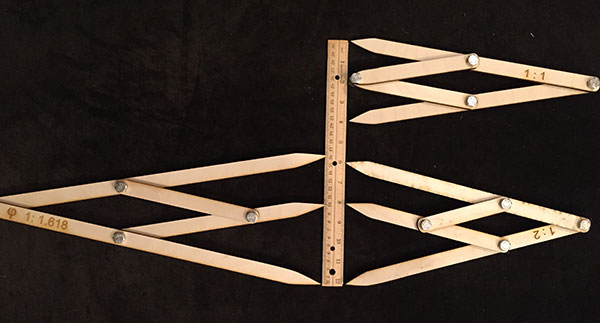Mirror– The grid printed on the mirror has lines in proportion to the golden ratio and it can be used to check your own face for Golden proportions.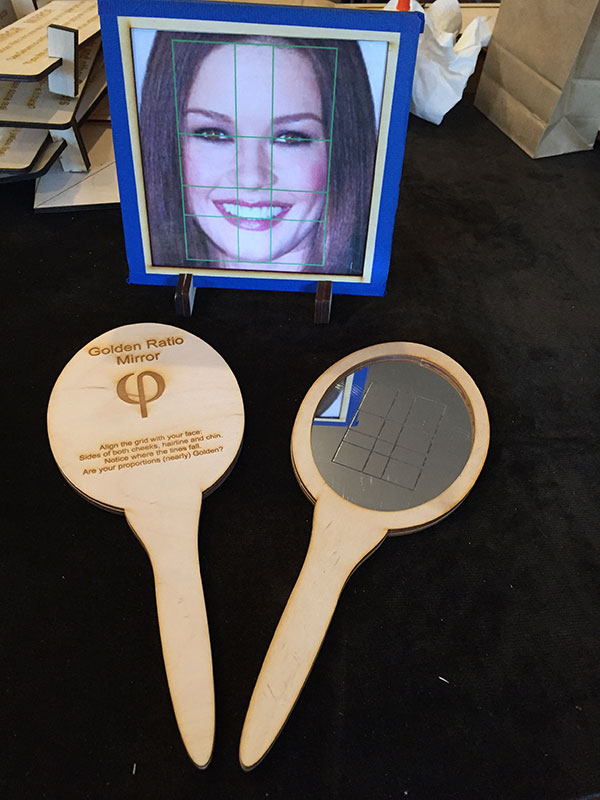Elbow – Wrist – Finger Measure. This gadget allows you to mark body parts – elbow, wrist and end of finger. After they are marked, you can use the calipers to check for Golden proportions.Ant Picture-Find the Golden proportions in this close up picture of an ant. If there are real specimens available, this would be the most powerful demonstration of the Golden Ratio in nature.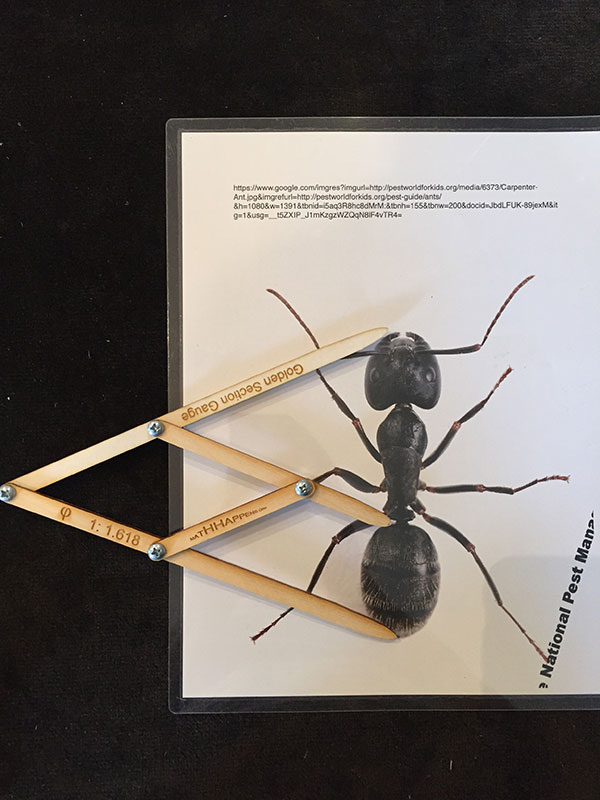Zebra Picture – highest impact would be if this were a picture of an actual zebra at your facility. It is a nice image because there are lots of ways to find the Golden Ratio.